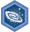## FANDOM

1,895 Pages

5th grade (U.S.): Place value and decimalsDescription
Achieve mastery in all skills in 5th grade (U.S.): Place value and decimals
Details
TypeEnergy point(s) awarded 0
Status Available
Disclosed?
Repeating?

This page contains exercises which pertain to the subcategory 5th grade (U.S.): Place value and decimals. Mastering them awards user with the Challenge Patch 5th grade (U.S.): Place value and decimals as well.

All items (16)

C
•Comparing decimal place value
•Comparing decimals challenge
F
•Fraction-decimal intuition
M
•Money and decimal place value intuition
•Multiplying and dividing decimals by powers of 10
•Multiplying and dividing whole numbers by powers of 10
O
•Ordering decimals
P
•Powers of ten
R
•Regrouping decimals
•Regrouping whole numbers
•Rewriting fractions as decimals
•Rounding decimals
•Rounding decimals 2
•Rounding whole numbers 2
U
•Understanding moving the decimal
W
•Writing and interpreting decimals